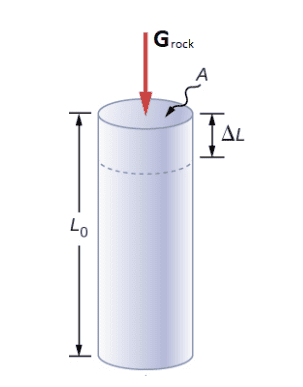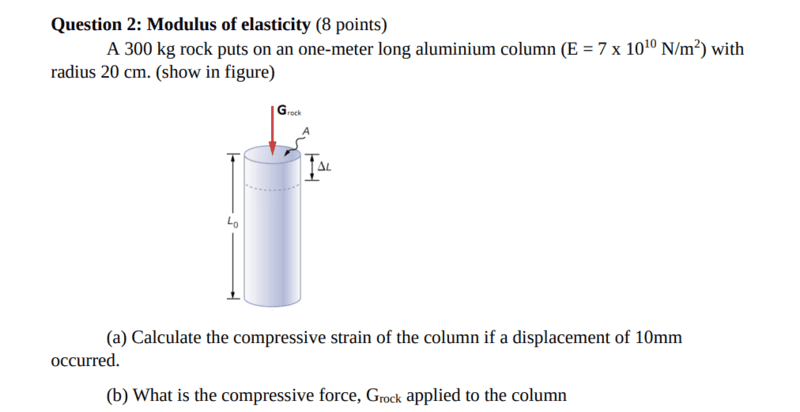# Basic Stress and Strain question -- Rock on top of a vertical column

• Hamiltonian
GPa) a compressive force of ##F = 2940N##.In summary, the column is displaced by 10μm due to the weight of the rock.f

#### Hamiltonian

Homework Statement
A ##300## kg rock puts on an one-meter long aluminium column (##E = 7 * 10^{10} N/m^2##) with radius ##20## cm. (show in figure)

(a) Calculate the compressive strain of the column if a displacement of ##10##mm
occurred.

(b) What is the compressive force, ##G_{rock}## applied to the column
Relevant Equations
##\sigma = E\epsilon## and ##\epsilon = \frac{\Delta L}{L}##a) I can find the compressive strain on the aluminium column using the formula ##\sigma = E\epsilon## as we know ##\sigma = F/A##. The area of the column is ##A = \pi r^2 = 0.126m^2## and the force on the column is ##F = 300*(9.8)N = 2940N##. The stress therefore is ##\sigma = \frac{2940N}{0.126m^2} = 23333.333N/m^2##

hence plugging the stress(##\sigma##) and the Modulus of Elacticity(E) into our original equation we find the strain, ##\epsilon = \frac{\sigma}{E} = \frac{23333.33}{7*10^{10}} = 3333.332857*10^{-10}##.

But if we use the definition of strain ##\epsilon = \frac{\Delta L}{L}## we get ##\epsilon = \frac{0.01m}{1m} = 0.01##. The compressive strain obviously can't have two different values, so where have I gone wrong in my reasoning?

b) Also Just to be safe the compressive force ##G_{rock}## applied to the column would be the weight of the rock i.e., ##300g N = 2940N##?

•Chestermiller
Seems like they over constrained the problem to me.

•Chestermiller
Seems like they over constrained the problem to me.
are you saying there's a mistake in the question?

are you saying there's a mistake in the question?
If you are given a modulus of elasticity and a strain, then the stress is fixed.

If they were intending for you to calculate ##G_{rock}## they bungled it when they gave its weight, and the deflection (and other relevant properties) it caused. Deflection is given by

$$\epsilon = \frac{ \delta}{L} = \frac{P\cancel{L}}{\cancel{L}AE}$$

You have everything on both sides, and if you did it the math correctly (which I didn't check as a yet) they don't match...

a) the compressive strain = the displacement under force.
This was given and does not need to be computed !

•nasu
a) the compressive strain = the displacement under force.
This was given and does not need to be computed !
Well it needs to be calculated as has been done in the following:

But if we use the definition of strain ##\epsilon = \frac{\Delta L}{L}## we get ##\epsilon = \frac{0.01m}{1m} = 0.01##. The compressive strain obviously can't have two different values, so where have I gone wrong in my reasoning?

a) the compressive strain = the displacement under force.
This was given and does not need to be computed !
No, it is the displacement divided by the total length.

•scottdave
are you saying there's a mistake in the question?
Not only is it overconstrained but the numbers are in violent disagreement.
A 10mm displacement is huge. Maybe it should be 10μm? That still leaves a factor of 30 to be found somewhere.

•erobz
so what do you reckon I do now? This problem is on my homework and I need to put one of the answers which one should I put.

The modulus method should equal the compression displacement method which is actual for low levels , which this is.

I cannot imagine 300 kg doing 10mm compression on a 40 mm diameter aluminum column and this is faulty data.

——-
To compute the compression of the aluminum column, we need to use the following formula:

δ = (F * L) / (A * E)

where:

• δ is the compression of the column
• F is the compressive force applied to the column
• L is the original length of the column
• A is the cross-sectional area of the column
• E is the modulus of elasticity of aluminum
From the problem statement, we have:

• Original length of the column, L = 1 m
• Diameter of the column, d = 40 mm = 0.04 m, so the radius r = d/2 = 0.02 m
• Cross-sectional area of the column, A = π * r^2 = 3.14 * (0.02)^2 = 0.001256 m^2
• Modulus of elasticity of aluminum, E = 70 GPa = 70 * 10^9 N/m^2
• Compressive force applied to the column, F = 400 kg = 400 * 9.8 N = 3920 N
Now we can substitute the values into the formula to obtain:

δ = (3920 N * 1 m) / (0.001256 m^2 * 70 * 10^9 N/m^2) = 0.000005788 m = 5.788 μm

Therefore, the compression of the aluminum column is 5.788 μm.

so what do you reckon I do now? This problem is on my homework and I need to put one of the answers which one should I put.
Are you sure it gives the displacement as 10mm, not 10μm?
Are you saying it is multiple choice? If so, what are the options?

Are you sure it gives the displacement as 10mm, not 10μm?
Are you saying it is multiple choice? If so, what are the optionsits 10mm.
It's not multiple choice.

Maybe we are not on Earth anymore.

View attachment 325359
its 10mm.
It's not multiple choice.
Then I give up. Even the grammar is faulty. What book is this?

•Chestermiller
Diameter of the column, d = 40 mm = 0.04 m, so the radius r = d/2 = 0.02 m
Cross-sectional area of the column, A = π * r^2 = 3.14 * (0.02)^2 = 0.001256 m^2
##20~\rm{cm} = 0.2 ~\rm{m}##

Homework Statement: A ##300## kg rock puts on an one-meter long aluminium column (##E = 7 * 10^{10} N/m^2##) with radius ##20## cm. (show in figure)

Also, the mass of the rock is ##300 ~\rm{kg}##, not ##400 ~\rm{kg}##.

Compressive force applied to the column, F = 400 kg = 400 * 9.8 N = 3920 N

Last edited:
•Lnewqban
Then I give up. Even the grammar is faulty. What book is this?
It's actually on my homework problem set for a physics class meant for cs majors. This is what happens when the computer science department tries to do physics:/

Everyone makes errors, even String Theorists and ChatGPT.

e.g. Diameter of the column, d = 40 mm = 0.04 m, so the radius r = d/2 = 0.02 m

But look at who makes the big bucks? What's wrong with this picture?Diameter of the column, d = 40 mm
Why are you repeating that claim?
Homework Statement: …. with radius ##20## cm.

sorry my err pls delete.